Download Presentation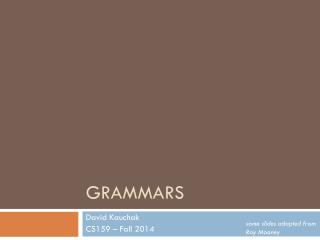Grammars

# Grammars - PowerPoint PPT Presentation

Grammars. David Kauchak CS159 – Fall 2014. some slides adapted from Ray Mooney. Admin. Assignment 3 Quiz #1 How was the lab last Thursday?. Simplified View of Linguistics. Phonetics. / waddyasai /.I am the owner, or an agent authorized to act on behalf of the owner, of the copyrighted work described.
Download Presentation## Grammars

An Image/Link below is provided (as is) to download presentation

Download Policy: Content on the Website is provided to you AS IS for your information and personal use and may not be sold / licensed / shared on other websites without getting consent from its author.While downloading, if for some reason you are not able to download a presentation, the publisher may have deleted the file from their server.

- - - - - - - - - - - - - - - - - - - - - - - - - - E N D - - - - - - - - - - - - - - - - - - - - - - - - - -
Presentation Transcript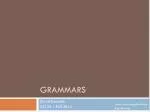### Grammars

David Kauchak

CS159 – Fall 2014

some slides adapted from Ray MooneyAssignment 3

Quiz #1

How was the lab last Thursday?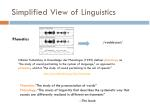Simplified View of Linguistics

Phonetics

Nikolai Trubetzkoy in Grundzüge der Phonologie (1939) defines phonology as "the study of sound pertaining to the system of language," as opposed to phonetics, which is "the study of sound pertaining to the act of speech."

http://en.wikipedia.org/wiki/Phonology

Phonetics: “The study of the pronunciation of words”

Phonology: “The areas of linguistics that describes the systematic way that sounds are differently realized in different environments”

--The book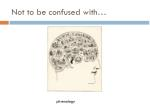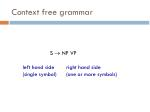Context free grammar

S  NP VP

left hand side

(single symbol)

right hand side

(one or more symbols)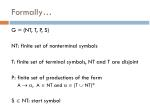Formally…

G = (NT, T, P, S)

NT: finite set of nonterminal symbols

T: finite set of terminal symbols, NT and T are disjoint

P: finite set of productions of the form

A  , A  NT and   (T  NT)*

S  NT: start symbol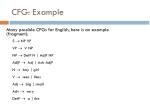CFG: Example

Many possible CFGs for English, here is an example (fragment):

S  NP VP

VP  V NP

NP DetP N | AdjP NP

N  boy | girl

V  sees | likes

Adj big | small

DetP a | the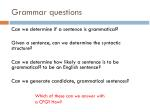Grammar questions

Can we determine if a sentence is grammatical?

Given a sentence, can we determine the syntactic structure?

Can we determine how likely a sentence is to be grammatical? to be an English sentence?

Can we generate candidate, grammatical sentences?

Which of these can we answer with a CFG? How?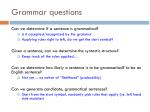Grammar questions

Can we determine if a sentence is grammatical?

• Is it accepted/recognized by the grammar
• Applying rules right to left, do we get the start symbol?

Given a sentence, can we determine the syntactic structure?

• Keep track of the rules applied…

Can we determine how likely a sentence is to be grammatical? to be an English sentence?

• Not yet… no notion of “likelihood” (probability)

Can we generate candidate, grammatical sentences?

• Start from the start symbol, randomly pick rules that apply (i.e. left hand side matches)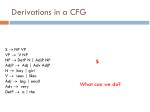Derivations in a CFG

S  NP VP

VP  V NP

NP  DetP N | AdjP NP

N  boy | girl

V  sees | likes

Adj  big | small

DetP  a | the

S

What can we do?Derivations in a CFG

S  NP VP

VP  V NP

NP  DetP N | AdjP NP

N  boy | girl

V  sees | likes

Adj  big | small

DetP  a | the

SDerivations in a CFG

S  NP VP

VP  V NP

NP  DetP N| AdjP NP

N  boy | girl

V  sees | likes

Adj  big | small

DetP  a | the

NP VP

What can we do?Derivations in a CFG

S  NP VP

VP  V NP

NP  DetP N | AdjP NP

N  boy | girl

V  sees | likes

Adj  big | small

DetP  a | the

NP VPDerivations in a CFG

S  NP VP

VP  V NP

NP  DetP N | AdjP NP

N  boy | girl

V  sees | likes

Adj  big | small

DetP  a | the

DetP N VPDerivations in a CFG

S  NP VP

VP  V NP

NP  DetP N | AdjP NP

N  boy| girl

V  sees | likes

Adj  big | small

DetP  a | the

DetP N VPDerivations in a CFG

S  NP VP

VP  V NP

NP DetP N | AdjP NP

N  boy | girl

V  sees | likes

Adj big | small

DetP a | the

the boy VPDerivations in a CFG

S  NP VP

VP  V NP

NP  DetP N| AdjP NP

N  boy | girl

V  sees | likes

Adj  big | small

DetP  a| the

the boy likes NPDerivations in a CFG

S  NP VP

VP  V NP

NP  DetP N | AdjP NP

N  boy | girl

V  sees | likes

Adj  big | small

DetP  a | the

the boy likes a girlDerivations in a CFG;Order of Derivation Irrelevant

S  NP VP

VP  V NP

NP  DetP N | AdjP NP

N  boy | girl

V  sees | likes

Adj  big | small

DetP  a | the

NP VP

DetP N VP

NP V NP

the boy likes a girlDerivations of CFGs

String rewriting system: we derive a string

Derivation history shows constituent tree:

S

NP

VP

DetP

N

the boy likes a girl

V

NP

the

boy

likes

N

DetP

girl

aParsing

Parsing is the field of NLP interested in automatically determining the syntactic structure of a sentence

parsing can be thought of as determining what sentences are “valid” English sentences

As a by product, we often can get the structureParsing

Given a CFG and a sentence, determine the possible parse tree(s)

I eat sushi with tuna

S -> NP VP

NP -> N

NP -> PRP

NP -> N PP

VP -> V NP

VP -> V NP PP

PP -> IN N

PRP -> I

V -> eat

N -> sushi

N -> tuna

IN -> with

What parse trees are possible for this sentence?

How did you do it?

What if the grammar is much larger?Parsing

S -> NP VP

NP -> PRP

NP -> N PP

NP -> N

VP -> V NP

VP -> V NP PP

PP -> IN N

PRP -> I

V -> eat

N -> sushi

N -> tuna

IN -> with

S

S

VP

VP

NP

NP

NP

PP

NP

PP

PRP

V

N

IN

N

PRP

V

N

IN

N

I eat sushi with tuna

I eat sushi with tuna

What is the difference between these parses?Parsing ambiguity

S -> NP VP

NP -> PRP

NP -> N PP

NP -> N

VP -> V NP

VP -> V NP PP

PP -> IN N

PRP -> I

V -> eat

N -> sushi

N -> tuna

IN -> with

S

S

VP

VP

NP

NP

NP

PP

NP

PP

PRP

V

N

IN

N

PRP

V

N

IN

N

I eat sushi with tuna

I eat sushi with tuna

How can we decide between these?A Simple PCFG

Probabilities!Just like n-gram language modeling, PCFGs break the sentence generation process into smaller steps/probabilities

The probability of a parse is the product of the PCFG rulesWhat are the different interpretations here?

Which do you think is more likely?= 1.0 * 0.1 * 0.7 * 1.0 * 0.4 * 0.18

* 1.0 * 1.0 * 0.18

= 0.0009072

= 1.0 * 0.1 * 0.3 * 0.7 * 1.0 * 0.18

* 1.0 * 1.0 * 0.18

= 0.0006804Parsing problems

Pick a model

• e.g. CFG, PCFG, …

Train (or learn) a model

• What CFG/PCFG rules should I use?
• Parameters (e.g. PCFG probabilities)?
• What kind of data do we have?

Parsing

• Determine the parse tree(s) given a sentenceSupervised

PCFG

Training

S →NP VP

S → VP

NP → Det A N

NP → NP PP

NP → PropN

A → ε

A → Adj A

PP → Prep NP

VP → V NP

VP → VP PP

0.9

0.1

0.5

0.3

0.2

0.6

0.4

1.0

0.7

0.3

S

S

NP VP

NP VP

John V NP PP

John V NP PP

put the dog in the pen

put the dog in the pen

English

PCFG: Training

If we have example parsed sentences, how can we learn a set of PCFGs?

Tree Bank

.

.

.Extracting the rules

S

S  NP VP

NP  PRP

PRP  I

VP  V NP

V  eat

NP  N PP

N  sushi

PP  IN N

IN  with

N  tuna

VP

NP

NP

PP

PRP

V

N

IN

N

I eat sushi with tuna

What CFG rules occur in this tree?Estimating PCFG Probabilities

We can extract the rules from the trees

S  NP VP

NP PRP

PRP I

VP  V NP

V  eat

NP  N PP

N  sushi

• S  NP VP 1.0
• VP  V NP 0.7
• VP  VP PP 0.3
• PP  P NP 1.0
• P  with 1.0
• V  saw 1.0

How do we go from the extracted CFG rules to PCFG rules?Estimating PCFG Probabilities

Extract the rules from the trees

Calculate the probabilities using MLEEstimating PCFG Probabilities

Occurrences

S NP VP 10

S  V NP 3

S  VP PP 2

NP  N 7

NP  N PP 3

NP  DT N 6

P( S  V NP) = ?

count(S  V NP)

3

=

P( S  V NP) = P( S  V NP | S) =

15

count(S)Grammar Equivalence

What does it mean for two grammars to be equal?Grammar Equivalence

Weak equivalence: grammars generate same set of strings

• Grammar 1: NP  DetP N and DetP a | the
• Grammar 2: NP  a N | the N

Strong equivalence: grammars have same set of derivation trees

• With CFGs, possible only with useless rules
• Grammar 2: NP  a N | the N
• Grammar 3: NP  a N | the N, DetP manyNormal Forms

There are weakly equivalent normal forms (Chomsky Normal Form, Greibach Normal Form)

A CFG is in Chomsky Normal Form (CNF) if all productions are of one of two forms:

• A  BC with A, B, Cnonterminals
• A a, with A a nonterminal and a a terminal

Every CFG has a weakly equivalent CFG in CNFCNF Grammar

S -> VP

VP -> VB NP

VP -> VP2 PP

VP2 -> VB NP

NP -> DT NN

NP -> NN

NP -> NP PP

PP -> IN NP

DT -> the

IN -> with

VB -> film

VB -> trust

NN -> man

NN -> film

NN -> trust

S -> VP

VP -> VB NP

VP -> VB NP PP

NP -> DT NN

NP -> NN

NP -> NP PP

PP -> IN NP

DT -> the

IN -> with

VB -> film

VB -> trust

NN -> man

NN -> film

NN -> trustProbabilistic Grammar Conversion

Original Grammar

Chomsky Normal Form

S → NP VP

S → X1 VP

X1 → Aux NP

S → book | include | prefer

0.01 0.004 0.006

S → Verb NP

S → VP PP

NP → I | he | she | me

0.1 0.02 0.02 0.06

NP → Houston | NWA

0.16 .04

NP → Det Nominal

Nominal → book | flight | meal | money

0.03 0.15 0.06 0.06

Nominal → Nominal Noun

Nominal → Nominal PP

VP → book | include | prefer

0.1 0.04 0.06

VP → Verb NP

VP → VP PP

PP → Prep NP

S → NP VP

S → Aux NP VP

S → VP

NP → Pronoun

NP → Proper-Noun

NP → Det Nominal

Nominal → Noun

Nominal → Nominal Noun

Nominal → Nominal PP

VP → Verb

VP → Verb NP

VP → VP PP

PP → Prep NP

0.8

0.1

0.1

0.2

0.2

0.6

0.3

0.2

0.5

0.2

0.5

0.3

1.0

0.8

0.1

1.0

0.05

0.03

0.6

0.2

0.5

0.5

0.3

1.0States

Helena (Montana)

What is the capitol of this state?Grammar questions

Can we determine if a sentence is grammatical?

Given a sentence, can we determine the syntactic structure?

Can we determine how likely a sentence is to be grammatical? to be an English sentence?

Can we generate candidate, grammatical sentences?

Next time:

parsing